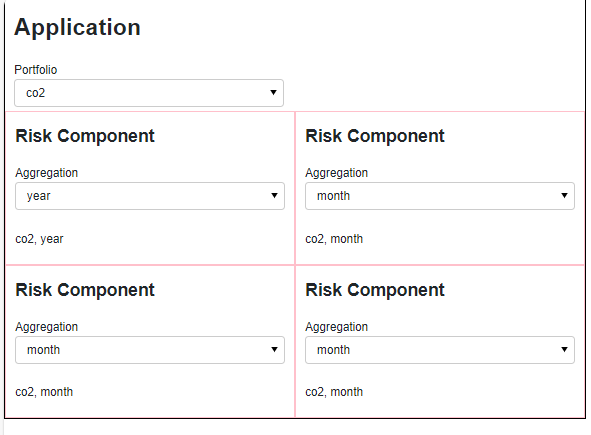# How to let application state flow to component state?

In order to be able to maintain and further develop my apps I want to split them into seperate, reusable components.

I’m often faced with the question of how to do this and how to let shared application state flow down to the components (or up). These concepts of state and flows are very discussed and supported in front languages like React. But its not clear to me how this should be done with Panel.

So my question is really how to do this. To help the discussion lets take a concrete example with state flowing down into components.

The application below has a shared `portfolio` state and some components that have their own `aggregation` state. When the `portfolio` state changes this flow down to the components as an update to their `portfolio` state. But the `aggregation` state of the components is not changed.

``````import panel as pn
import param

pn.extension()

class RiskComponent(pn.viewable.Viewer):
portfolio = param.String()
aggregation = param.Selector(default="month", objects=["month", "year"])

def __panel__(self):
return pn.Column("## Risk Component", self.param.aggregation, self._get_risk, styles={"border": "1px solid pink"})

@pn.depends("portfolio", "aggregation")
def _get_risk(self):
return f"{self.portfolio}, {self.aggregation}"

class Application(pn.viewable.Viewer):
portfolio = param.Selector(default="power", objects=["power", "gas", "co2"])

def _get_bound_risk(self):
risk = RiskComponent(portfolio=self.portfolio)
def _get_updated_component(component, **params):
component.param.update(params)
return component
return pn.bind(_get_updated_component, component=risk, portfolio=self.param.portfolio)

def __panel__(self):

return pn.Column(
"# Application",
self.param.portfolio,
pn.Row(self._get_bound_risk(),self._get_bound_risk(),),
pn.Row(self._get_bound_risk(),self._get_bound_risk(),),
styles={"border": "2px solid black"}
)

Application().servable()
``````I like everything about the above python code except the function `get_bound_risk`. It is just boiler plate code. How can I replace `_get_bound_risk` function with one line of code?

## Update

I can shorten via

``````def _get_bound_risk(self):
risk = RiskComponent(portfolio=self.portfolio)
pn.bind(risk.param.update, portfolio=self.param.portfolio, watch=True)
return risk
``````

But its still 3 lines of code instead of 1The below is what I want. But it does not work

``````def _get_bound_risk(self):
return RiskComponent(portfolio=self.param.portfolio)
``````

I can do this with Panel components. Why can I not do this with Parameterized classes in general?

## Solution

I can do this now with param 2.0 using `allow_refs`.

This is HUGE. Thanks

``````import panel as pn
import param

pn.extension()

class RiskComponent(pn.viewable.Viewer):
portfolio = param.String(allow_refs=True)
aggregation = param.Selector(default="month", objects=["month", "year"])

def __panel__(self):
return pn.Column("## Risk Component", self.param.aggregation, self._get_risk, styles={"border": "1px solid pink"})

@pn.depends("portfolio", "aggregation")
def _get_risk(self):
return f"{self.portfolio}, {self.aggregation}"

class Application(pn.viewable.Viewer):
portfolio = param.Selector(default="power", objects=["power", "gas", "co2"])

def __panel__(self):
return pn.Column(
"# Application",
self.param.portfolio,
pn.Row(RiskComponent(portfolio=self.param.portfolio),RiskComponent(portfolio=self.param.portfolio)),
pn.Row(RiskComponent(portfolio=self.param.portfolio),RiskComponent(portfolio=self.param.portfolio)),
styles={"border": "2px solid black"}
)

Application().servable()
``````
1 Like